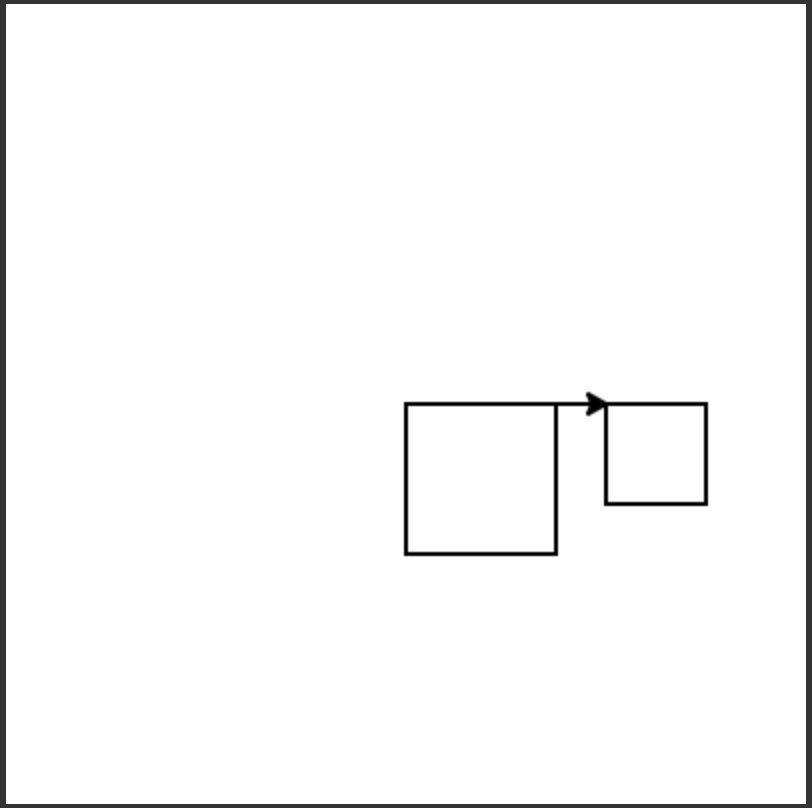# 5.6. Multiple Parameters¶

Below we have a program that draws a square of size 100. What if we want to draw a different size square, like one with a side length of 50? We could do that by changing each of the 100s into a 50.

But, this means we have to change each of the four forward statements and we could make a mistake and not set all of them to the same number. Is there a better way? What if we create a variable size and set its value to the amount to move forward?

Now the program is easier to change since we only have one line to change - size = 50 - to draw another size square. Once we change the size variable, all of the forward commands will use that new value.

But, the procedure can still only draw a square of one particular size. We can’t use it to draw two different size squares. If we want grace to draw a small square and then a large square, we would need two different procedures:

 1def square(turtle):
2    size = 50
3    turtle.forward(size)
4    turtle.right(90)
5    turtle.forward(size)
6    turtle.right(90)
7    turtle.forward(size)
8    turtle.right(90)
9    turtle.forward(size)
10    turtle.right(90)
11
12def largesSquare(turtle):
13    size = 100
14    turtle.forward(size)
15    turtle.right(90)
16    turtle.forward(size)
17    turtle.right(90)
18    turtle.forward(size)
19    turtle.right(90)
20    turtle.forward(size)
21    turtle.right(90)


Yuck. How repetitive. Now imagine we want to make squares of size 75 and 25 as well. We would need 4 different procedures that all basically look the same! Remember that if you find yourself writing the same code multiple times, there is probably a better way to do things.

In this case, we can add an additional parameter to the procedure that specifies the size of the square. Recall that a procedure can have as many parameters (inputs) as we like - just separate the names for the parameters with a comma like: (turtle, size).

Now that square has two parameters, any call to square must provide two arguments. We need to specify the name of the turtle to draw with, then the size of the square to draw. Something like square(grace, 100) or square(grace, 50).

The following code assumes that a procedure square has been defined that takes a size. The code should create a turtle and then use it to draw a square, move forward, and draw a second square as shown below, but the lines are mixed up.